0

# 【JavaSE|数据结构】堆，优先级队列，topK问题与堆排序

⭐️前面的话⭐️

📒博客主页：未见花闻的博客主页
🎉欢迎关注🔎点赞👍收藏⭐️留言📝
📌本文由未见花闻原创，CSDN首发！
📆首发时间：🌴2022年2月14日🌴
✉️坚持和努力一定能换来诗与远方！
💭参考书籍：📚《Java核心技术》，📚《Java编程思想》，📚《Effective Java》
💬参考在线编程网站：🌐牛客网🌐力扣

🙏作者水平很有限，如果发现错误，一定要及时告知作者哦！感谢感谢！

### 📌导航小助手📌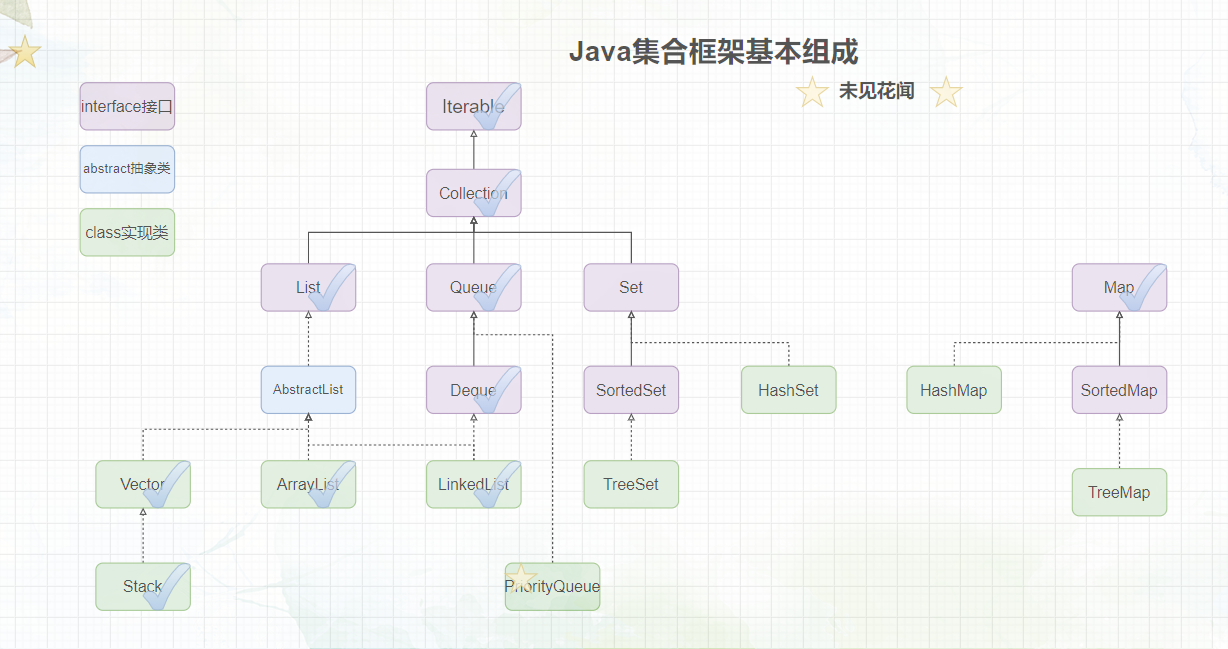## 1.1什么是堆？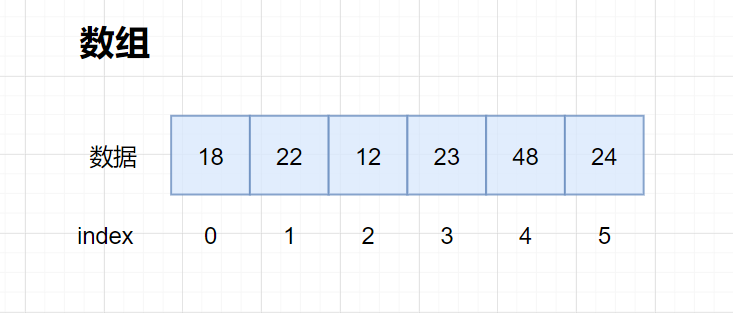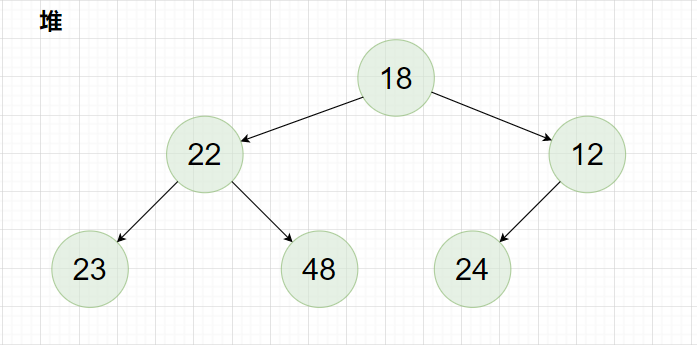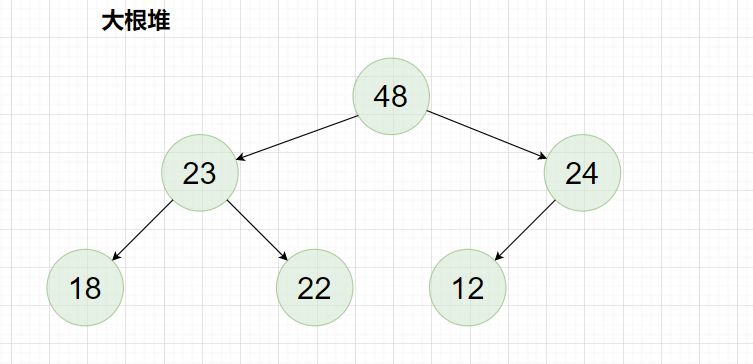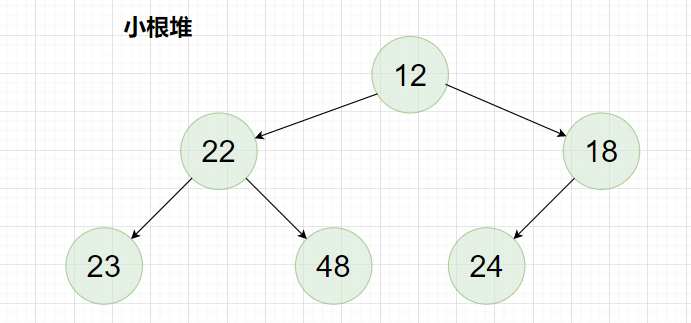## 1.2堆的创建

1. 找到堆中最后一个子树，该子树根结点不能为叶子结点。
2. 对该子树进行向下方向的调整，若该堆为大根堆，找到该树两个子结点值较大的结点与根结点进行比较，如果这个子结点比根结点要，则先交换两结点的值，然后在堆的大小范围内，对被交换结点的子树作相同的调整，直到被调整的子树下标超出堆的大小或满足大堆条件，反之如果堆为小根堆，找到该树两个子结点值较小的结点与根结点进行比较，如果这个子结点比根结点要，则先交换两结点的值，然后在堆的大小范围内，对被交换结点的子树作相同的调整，直到被调整的子树下标超出堆的大小或满足小堆条件。
3. 从最后一棵子树（该树不为叶子，最右边一棵高度为2的子树）开始，整棵树（以堆顶结点所代表的树）为结束，按此顺序对所有树进行向下调整，这个大（小）堆就创建好了。

``````parent
``````

，其左子树根结点为

``````child
``````

，堆大小为

``````len
``````

，由于该树是一棵完全二叉树，所以下标会满足

``````    c

h

i

l

d

=

2

∗

p

a

r

e

n

t

+

1

；

p

a

r

e

n

t

=

(

c

h

i

l

d

−

1

)

/

2

child=2*parent+1；parent=(child-1)/2

child=2∗parent+1；parent=(child−1)/2
``````

``````    l

e

n

−

1

len-1

len−1，父结点下标为

(

l

e

n

−

2

)

/

2

(len-2)/2

(len−2)/2。
``````

``````child++
``````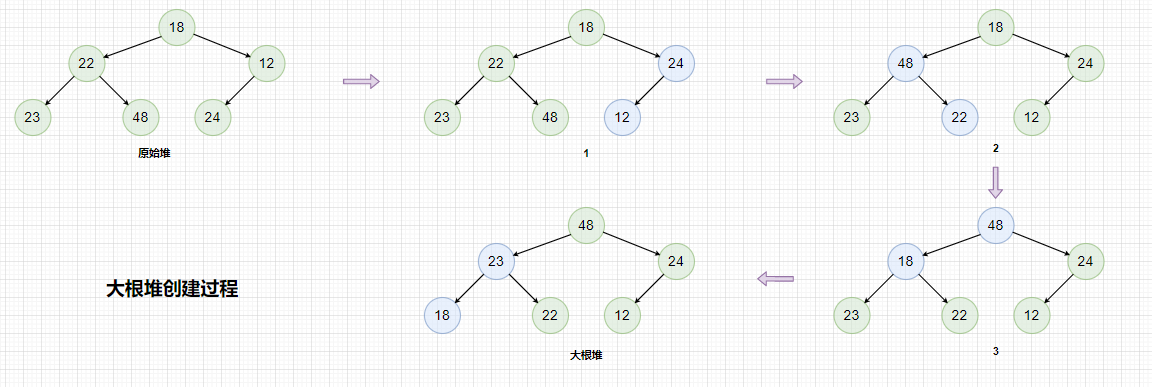``````publicclassHeap{privateint[] elem;privateint usedSize;publicHeap(){this.elem =newint;}//基本操作方法}
``````

``````/**
*
* @param arr 目标交换数组对象
* @param index1 下标1
* @param index2 下标2
*/privatevoidswap(int arr[],int index1,int index2){int tmp = arr[index1];
arr[index1]= arr[index2];
arr[index2]= tmp;}
``````
``````/**
* 向下调整
* @param parent 父节点下标
* @param len 堆大小
*/publicvoidshiftDown(int parent,int len){int child =2* parent +1;while(child < len){if(child +1< len &&this.elem[child]<this.elem[child +1]){
child++;}if(this.elem[child]>this.elem[parent]){swap(this.elem, child, parent);
parent = child;
child =2* parent +1;}else{break;}}}
``````
``````/**
* 创建大根堆
* @param data 堆中的数据
*/publicvoidcreatCopyBigHeap(int[] data){//拷贝数据for(int i =0; i < data.length; i++){if(isFull()){this.elem =Arrays.copyOf(this.elem,2*this.elem.length);}this.elem[i]= data[i];
usedSize++;}//从最后一棵完全二叉树子树开始调整int child =this.usedSize -1;int parent =(child -1)/2;while(parent >=0){shiftDown(parent, usedSize);
parent--;}}//或者直接利用数组对象，不对它进行深拷贝publicvoidcreatBigHeap(int[] data){//拷贝数据this.elem = data;
usedSize = data.length;//从最后一棵完全二叉树子树开始调整int child =this.usedSize -1;int parent =(child -1)/2;while(parent >=0){shiftDown(parent, usedSize);
parent--;}}
``````

``````h
``````

，堆元素个数为

``````n
``````

，建堆时需要对所有高度大于1的子树进行调整，最坏情况下该堆是一个满堆，设该堆所处层次为

``````x
``````

（从1开始），则第

``````x
``````

``````h-x
``````

``````     2

h

−

1

2^{h-1}

2h−1个结点，由于只调整高度大于1的子树，因此
``````
``````x
``````

``````    [

1

,

h

−

1

]

[1,h-1]

[1,h−1]。
``````

``````    S

S

S:
``````

（1）

``````     S

=

2

0

∗

(

h

−

1

)

+

2

1

∗

(

h

−

2

)

+

.

.

.

+

2

x

−

1

∗

(

h

−

x

)

+

.

.

.

+

2

h

−

2

∗

1

S=2^{0}*(h-1)+2^{1}*(h-2)+...+2^{x-1}*(h-x)+...+2^{h-2}*1

S=20∗(h−1)+21∗(h−2)+...+2x−1∗(h−x)+...+2h−2∗1
``````

``````    等

比

数

列

∗

等

差

数

列

等比数列*等差数列

``````
``````2
``````

（2）

``````     2

S

=

2

1

∗

(

h

−

1

)

+

2

2

∗

(

h

−

2

)

+

.

.

.

+

2

x

∗

(

h

−

x

)

+

.

.

.

+

2

h

−

1

∗

1

2S=2^{1}*(h-1)+2^{2}*(h-2)+...+2^{x}*(h-x)+...+2^{h-1}*1

2S=21∗(h−1)+22∗(h−2)+...+2x∗(h−x)+...+2h−1∗1
``````

（2）-（1）得:

``````     S

=

2

1

+

2

2

+

.

.

.

+

.

.

.

+

2

h

−

2

+

2

h

−

1

−

2

0

∗

(

h

−

1

)

S=2^{1}+2^{2}+...+...+2^{h-2}+2^{h-1}-2^{0}*(h-1)

S=21+22+...+...+2h−2+2h−1−20∗(h−1)
``````

``````h-1
``````

，等比数列求和公式为

``````     S

n

=

a

1

(

1

−

q

n

)

/

(

1

−

q

)

S_n=a_1(1-q^n)/(1-q)

Sn​=a1​(1−qn)/(1−q)。

S

=

2

∗

(

1

−

2

h

−

1

)

/

(

−

1

)

−

h

+

1

S=2*(1-2^{h-1})/(-1)-h+1

S=2∗(1−2h−1)/(−1)−h+1

S

=

2

h

−

h

−

1

S=2^h-h-1

S=2h−h−1
``````

``````    h

=

l

o

g

2

(

n

+

1

)

h=log_2(n+1)

h=log2​(n+1)

S

=

n

+

1

−

l

o

g

2

(

n

+

1

)

−

1

=

n

−

l

o

g

2

(

n

+

1

)

S=n+1-log_2(n+1)-1=n-log_2(n+1)

S=n+1−log2​(n+1)−1=n−log2​(n+1)
``````

## 2.2PriorityQueue

PriorityQueue构造方法：

``````importjava.util.Comparator;importjava.util.PriorityQueue;publicclassTest{publicstaticvoidmain(String[] args){PriorityQueue<Integer> priorityQueue1 =newPriorityQueue<>();//创建基于小根堆的优先队列
priorityQueue1.offer(1);
priorityQueue1.offer(2);
priorityQueue1.offer(3);System.out.println(priorityQueue1);System.out.println("============");PriorityQueue<Integer> priorityQueue2 =newPriorityQueue<>(newComparator<Integer>(){@Overridepublicintcompare(Integer o1,Integer o2){return o1 - o2;}});//使用隐藏内部类创建基于小根堆的优先队列
priorityQueue2.offer(1);
priorityQueue2.offer(2);
priorityQueue2.offer(3);System.out.println(priorityQueue2);System.out.println("============");PriorityQueue<Integer> priorityQueue3 =newPriorityQueue<>(newComparator<Integer>(){@Overridepublicintcompare(Integer o1,Integer o2){return o2 - o1;}});//使用隐藏内部类创建基于大根堆的优先队列
priorityQueue3.offer(1);
priorityQueue3.offer(2);
priorityQueue3.offer(3);System.out.println(priorityQueue3);System.out.println("============");PriorityQueue<Integer> priorityQueue4 =newPriorityQueue<>((x, y)-> x-y);//使用lambda表达式创建基于小根堆的优先队列
priorityQueue4.offer(1);
priorityQueue4.offer(2);
priorityQueue4.offer(3);System.out.println(priorityQueue4);System.out.println("============");PriorityQueue<Integer> priorityQueue5 =newPriorityQueue<>((x, y)-> y-x);//使用lambda表达式创建基于大根堆的优先队列
priorityQueue5.offer(1);
priorityQueue5.offer(2);
priorityQueue5.offer(3);System.out.println(priorityQueue5);System.out.println("============");}}
``````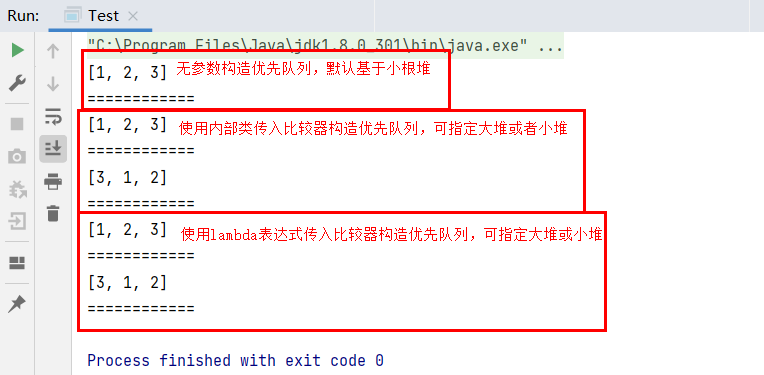PriorityQueue基本操作方法：

PriorityQueue其他常用方法：

## 2.3简单实现优先队列的基本操作

1. 将元素放入堆尾。
2. 对堆尾的结点进行向上方向调整，因为入队一个元素后，堆中只有一条到新插入结点路径上不是大根堆，所以仅需对该结点进行向上调整，所谓向上调整就是比较调整结点与父亲结点值的大小，以大根堆为例，如果该结点值比较大，则与父亲结点交换值，否则不需调整，该堆已经满足大根堆条件，因为交换后不知道上面的子树是否为大根堆，所以需要对交换路径上所有的结点进行相同的向上调整，直到调整完堆顶，过程中出现父亲结点比较大则结束调整，反之如果是小根堆，就把调整结点与父亲结点的比较方式改变一下即可。
3. 入队完成。

``````publicclassHeap{privateint[] elem;privateint usedSize;publicHeap(){this.elem =newint;}/**
*
* @param arr 目标交换数组对象
* @param index1 下标1
* @param index2 下标2
*/privatevoidswap(int arr[],int index1,int index2){int tmp = arr[index1];
arr[index1]= arr[index2];
arr[index2]= tmp;}//基本操作方法}
``````

``````//判断队列是否满或空publicbooleanisFull(){returnthis.elem.length ==this.usedSize;}publicbooleanidEmpty(){returnthis.usedSize ==0;}
``````
``````//向上调整privatevoidshiftUp(int child){int parent =(child -1)/2;while(child >0){if(this.elem[parent]<this.elem[child]){swap(this.elem, parent, child);
child = parent;
parent =(child -1)/2;}else{break;}}}
``````
``````/**
* 基于大堆的优先队列进队
*/publicvoidoffer(int val){if(isFull()){this.elem =Arrays.copyOf(this.elem,2*this.elem.length);}//1.将元素插入堆尾this.elem[usedSize++]= val;//2.重新调整为大堆：向上调整shiftUp(usedSize -1);}
``````

1. 将堆顶元素与堆尾元素交换，保存并删除堆尾元素。
2. 对堆顶元素向下调整，因为堆顶交换元素的路径可能会破坏大根堆（或小根堆）。
3. 返回之前保存的堆尾元素，即原优先队列队头元素。

``````/**
* 基于大堆的优先队列出队
* @return 堆顶元素
*/publicintpoll(){if(idEmpty()){thrownewRuntimeException("优先队列为空!");}//1.将堆顶元素与堆尾元素交换swap(this.elem,0, usedSize -1);//2.保存原堆顶元素的值，并删除堆中原堆顶元素的值int ret =this.elem[--usedSize];//3.调整堆顶为根结点的树，使其调整为大堆shiftDown(0, usedSize);return ret;}
``````

``````/**
* 获取优先队列（基于大堆）队头元素
* @return 堆顶元素
*/publicintpeek(){if(idEmpty()){thrownewRuntimeException("优先队列为空!");}returnthis.elem;}
``````

## 2.4堆排序

1. 将数组转换成大根堆，使用索引`end`标记最后一个元素。
2. 将堆顶元素与`end`处元素交换，`end--`
3. 对堆顶结点进行向下调整，调整的结点下标不大于`end`
4. 重复2,3步骤，直到`end<=0`

``````end
``````

``````end-1
``````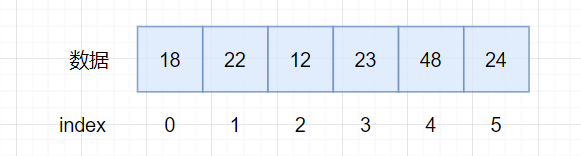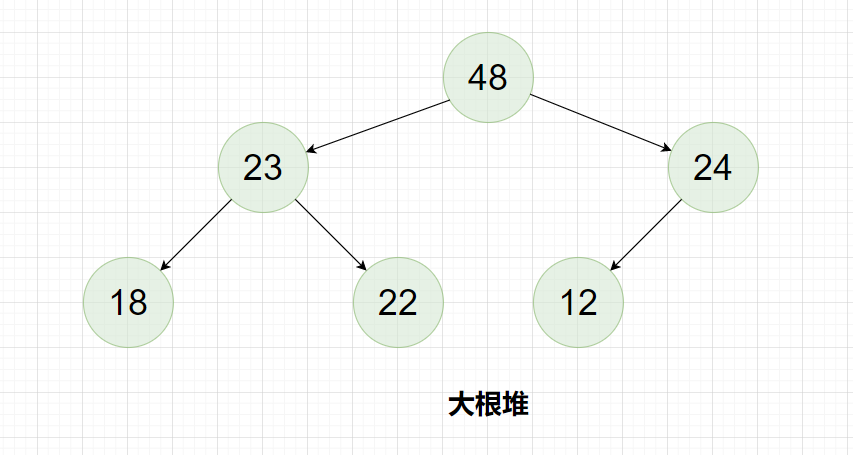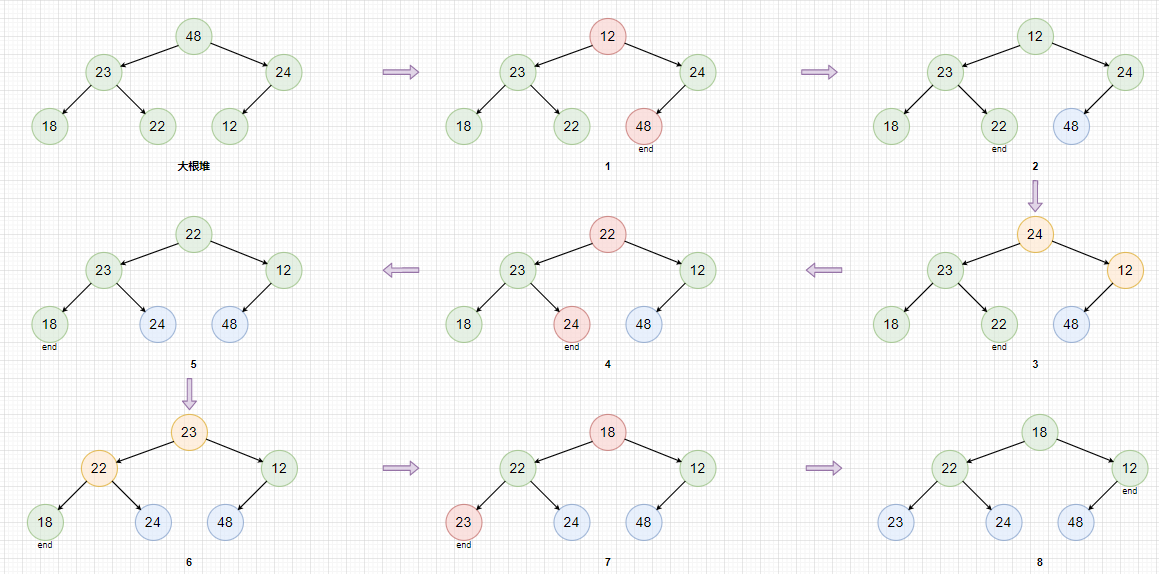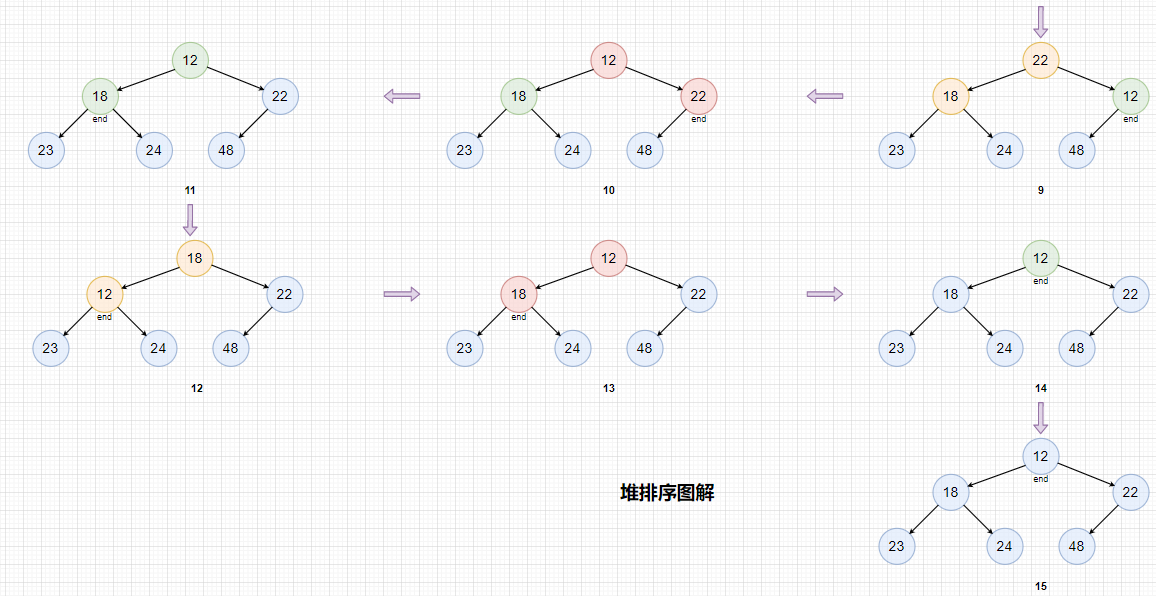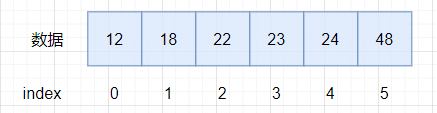``````publicclassHeap{privateint[] elem;privateint usedSize;publicHeap(){this.elem =newint;}/**
*
* @param arr 目标交换数组对象
* @param index1 下标1
* @param index2 下标2
*/privatevoidswap(int arr[],int index1,int index2){int tmp = arr[index1];
arr[index1]= arr[index2];
arr[index2]= tmp;}publicvoidcreatBigHeap(int[] data){//拷贝数据this.elem = data;
usedSize = data.length;//从最后一棵完全二叉树子树开始调整int child =this.usedSize -1;int parent =(child -1)/2;while(parent >=0){shiftDown(parent, usedSize);
parent--;}}/**
* 向下调整
* @param parent 父节点下标
* @param len 堆大小
*/publicvoidshiftDown(int parent,int len){int child =2* parent +1;while(child < len){if(child +1< len &&this.elem[child]<this.elem[child +1]){
child++;}if(this.elem[child]>this.elem[parent]){swap(this.elem, child, parent);
parent = child;
child =2* parent +1;}else{break;}}}}
``````

``````/**
* 堆排序
* @param arr 排序对象
* @return 返回排序好的对象
*/publicint[]heapSort(int[] arr){creatBigHeap(arr);int end = usedSize -1;for(int i = end; i >0; i--){swap(this.elem,0, i);shiftDown(0,i);}returnthis.elem;}
``````

## 3.TopK问题

topK问题指的是在一个很大数量级的数据中找出最大或者最小的前k个元素，比如在100万个数据中找到最大的10个数。

``````importjava.util.Comparator;importjava.util.PriorityQueue;publicclassTopK{/**
* 求数组中最大k个元素
* @param arr 数据
* @param k 最大元素个数
* @return 小根堆
*/publicstaticPriorityQueue<Integer>topKMax(int[] arr,int k){PriorityQueue<Integer> priorityQueue =newPriorityQueue<>();if(k <=0)return priorityQueue;for(int i =0; i < arr.length; i++){if(i < k){
priorityQueue.offer(arr[i]);}else{if(priorityQueue.isEmpty())return priorityQueue;int top = priorityQueue.peek();if(arr[i]> top){//1.堆顶出队
priorityQueue.poll();//2.入队并重新调整为小根堆
priorityQueue.offer(arr[i]);}}}return priorityQueue;}/**
* 求前k个最小的元素
* @param arr 数据
* @param k 最小的元素个数
* @return 大根堆
*/publicstaticPriorityQueue<Integer>topKMin(int[] arr,int k){//建大堆PriorityQueue<Integer> priorityQueue =newPriorityQueue<>(newComparator<Integer>(){@Overridepublicintcompare(Integer o1,Integer o2){return o2 - o1;}});if(k <=0)return priorityQueue;for(int i =0; i < arr.length; i++){if(i < k){
priorityQueue.offer(arr[i]);}else{if(priorityQueue.isEmpty())return priorityQueue;int top = priorityQueue.peek();if(arr[i]< top){//1.堆顶出队
priorityQueue.poll();//2.入队并重新调整为大根堆
priorityQueue.offer(arr[i]);}}}return priorityQueue;}publicstaticvoidmain(String[] args){int[] data ={3,2,4,5,6,1,7,9,8};PriorityQueue<Integer> ret =topKMax(data,3);//7,8,9System.out.print("前k个最大的元素：");System.out.println(ret);
ret =topKMin(data,3);System.out.print("前k个最小的元素：");System.out.println(ret);}}
``````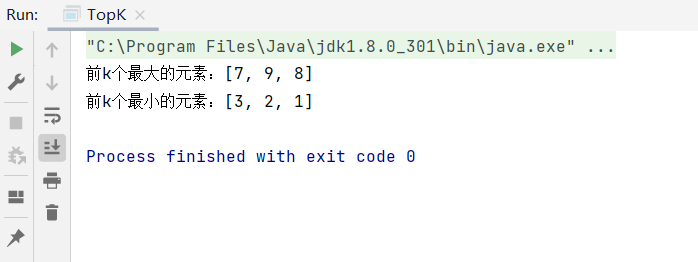373. 查找和最小的 K 对数字

``````nums1
``````

``````nums2
``````

, 以及一个整数

``````k
``````

``````(u,v)
``````

，其中第一个元素来自

``````nums1
``````

，第二个元素来自

``````nums2
``````

``````k
``````

``````(u1,v1)
``````

,

``````(u2,v2)
``````

``````(uk,vk)
``````

``````输入: nums1 = [1,7,11], nums2 = [2,4,6], k = 3

[1,2],[1,4],[1,6],[7,2],[7,4],[11,2],[7,6],[11,4],[11,6]
``````

``````输入: nums1 = [1,1,2], nums2 = [1,2,3], k = 2

[1,1],[1,1],[1,2],[2,1],[1,2],[2,2],[1,3],[1,3],[2,3]
``````

``````输入: nums1 = [1,2], nums2 = , k = 3

``````

• `1 <= nums1.length, nums2.length <= 105`
• `-109 <= nums1[i], nums2[i] <= 109`
• `nums1``nums2` 均为升序排列
• `1 <= k <= 1000`

💡解决思路：

``````k
``````

，用来存放前

``````k
``````

``````k
``````

``````min(k,len)
``````

``````len
``````

``````k
``````

``````k
``````

``````publicList<List<Integer>>kSmallestPairs(int[] nums1,int[] nums2,int k){//构造大根堆PriorityQueue<List<Integer>> priorityQueue =newPriorityQueue(newComparator<List<Integer>>(){@Overridepublicintcompare(List<Integer> o1,List<Integer> o2){return o2.get(0)+ o2.get(1)- o1.get(0)- o1.get(1);}});for(int i =0; i <Math.min(k,nums1.length); i++){for(int j =0; j <Math.min(k, nums2.length); j++){List<Integer> digits =newArrayList<>();
priorityQueue.offer(digits);}else{int topAdd = priorityQueue.peek().get(0)+ priorityQueue.peek().get(1);if(digits.get(0)+ digits.get(1)< topAdd){//1.出堆顶元素
priorityQueue.poll();//2.入目标元素
priorityQueue.offer(digits);}else{//3.因为两个数组升序排列，此时如果比堆顶元素大，则后面的组合必然比堆顶元素大，此时应该直接结束循环break;}}}}//返回值是List所以先得将优先队列转换成List再返回List<List<Integer>> ret =newArrayList(priorityQueue);return ret;}
``````

215. 数组中的第K个最大元素### “【JavaSE|数据结构】堆，优先级队列，topK问题与堆排序”的评论:

##### 关于作者##### overfit同步小助手